Successfully reported this slideshow.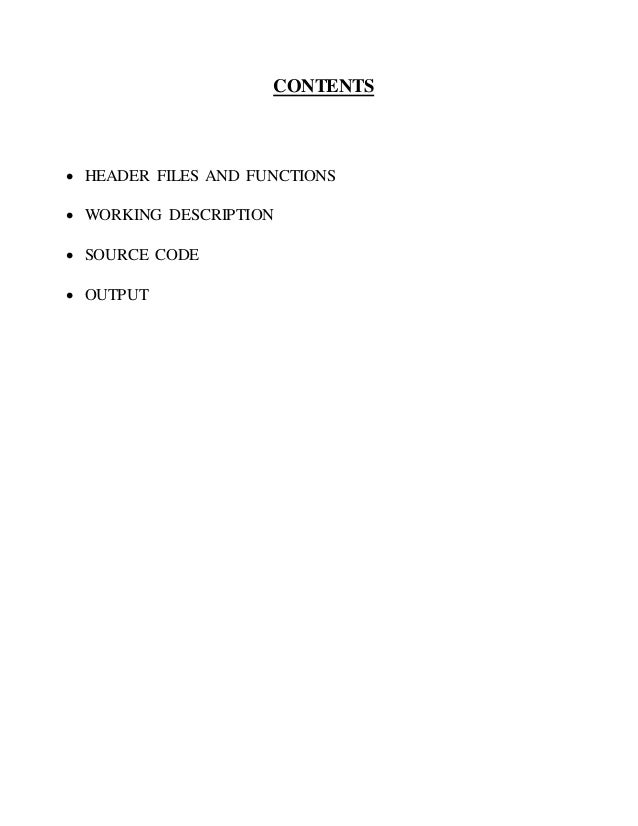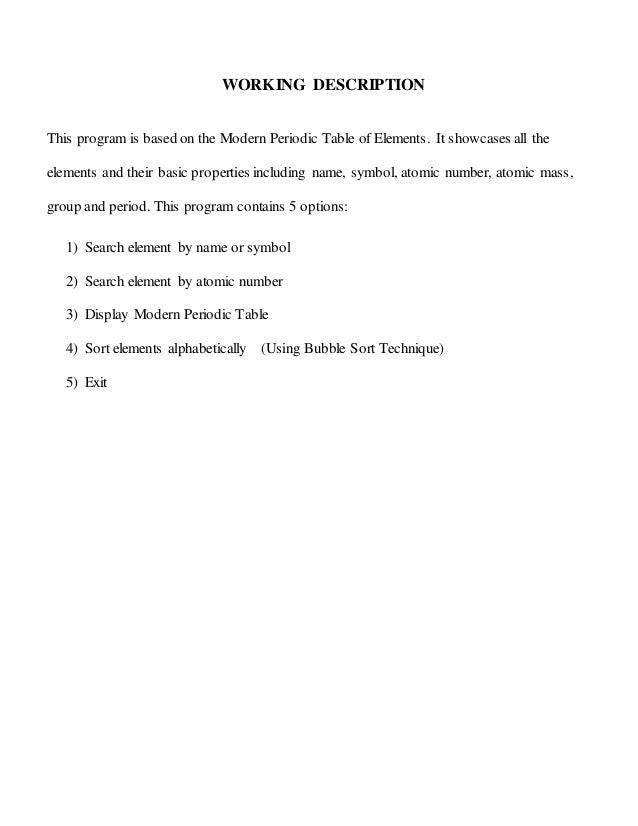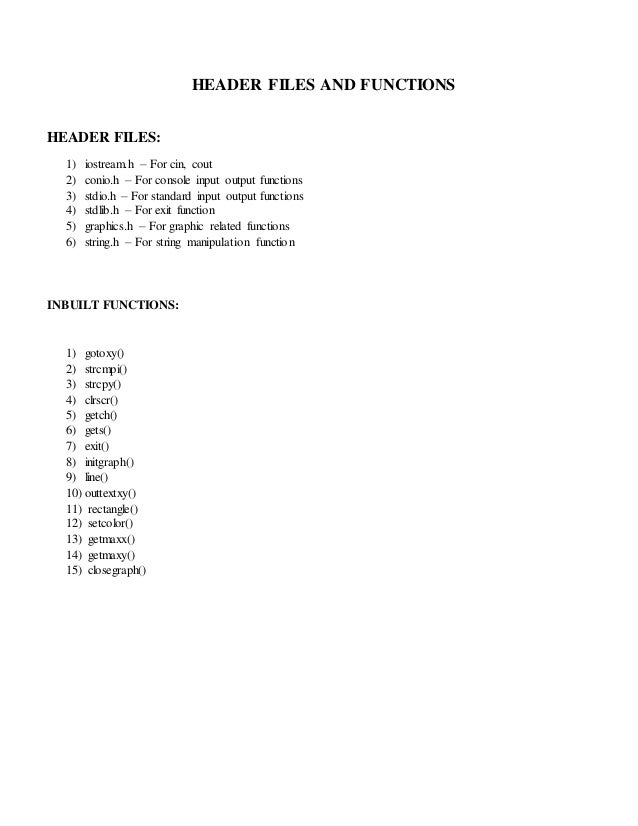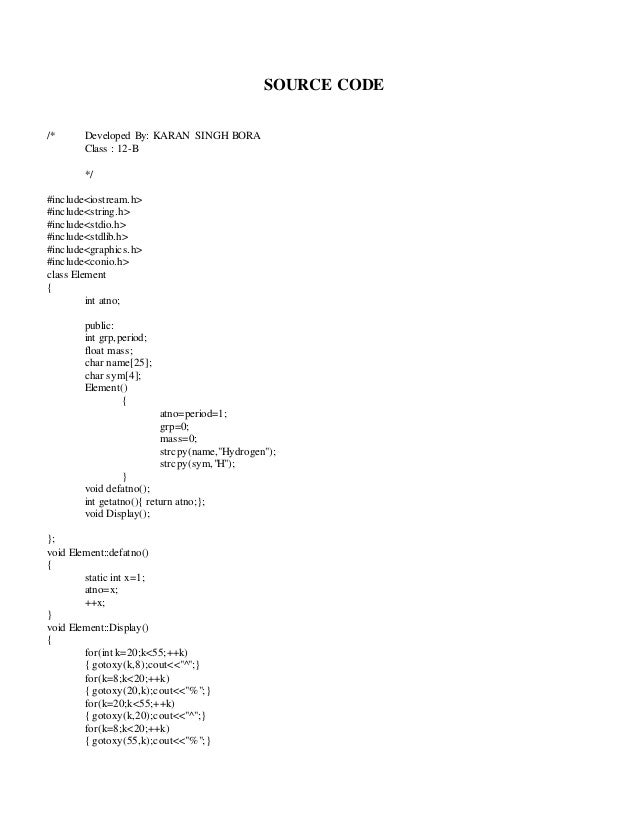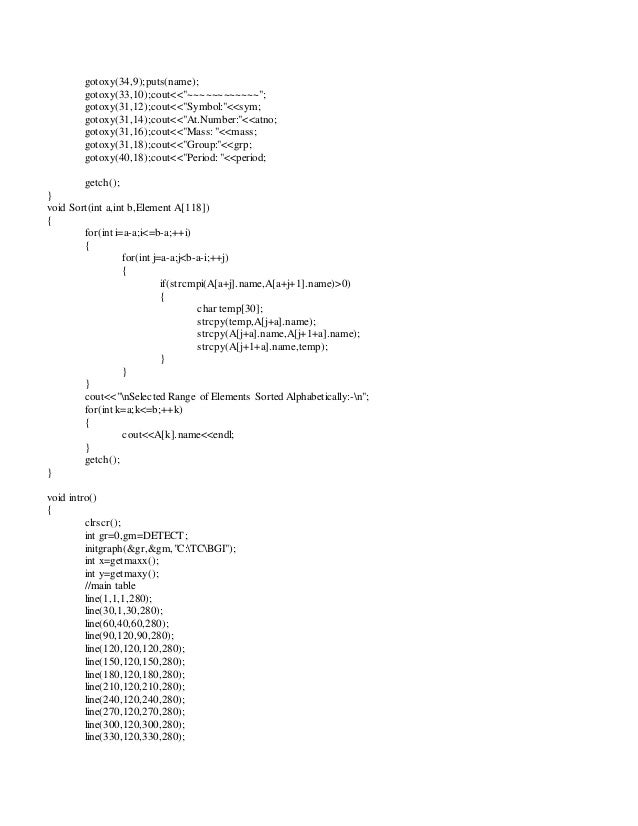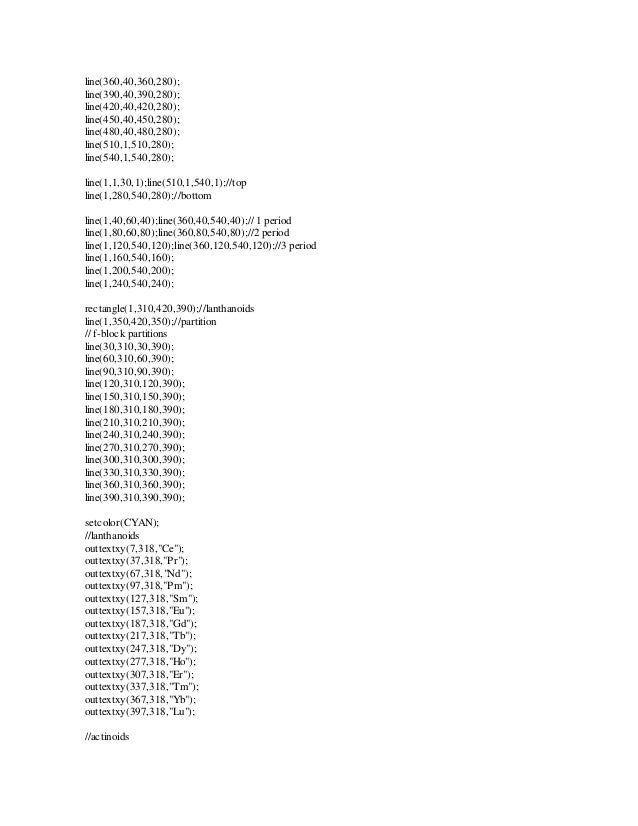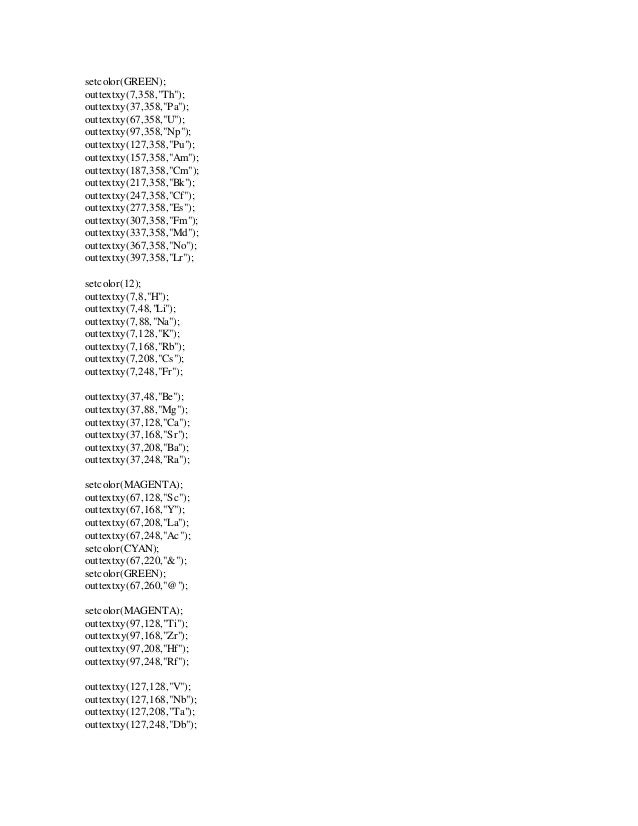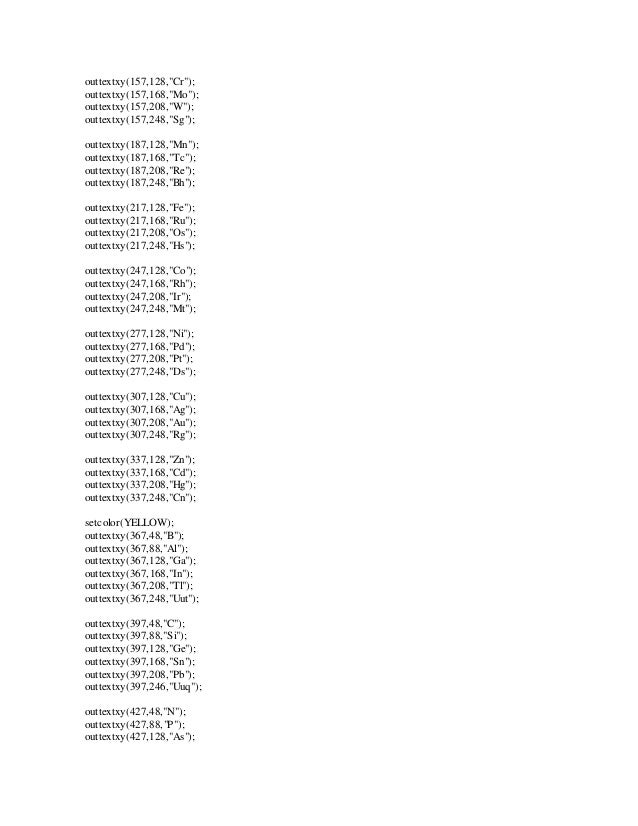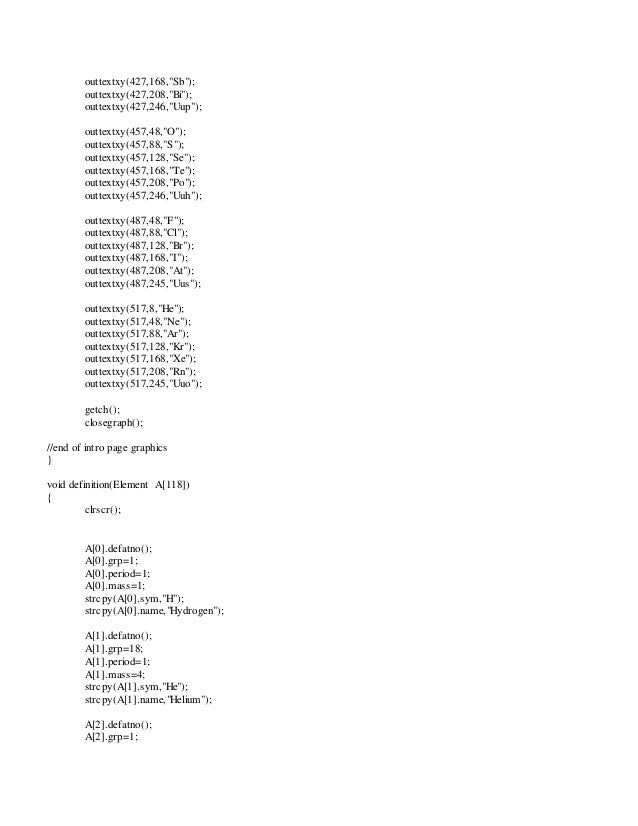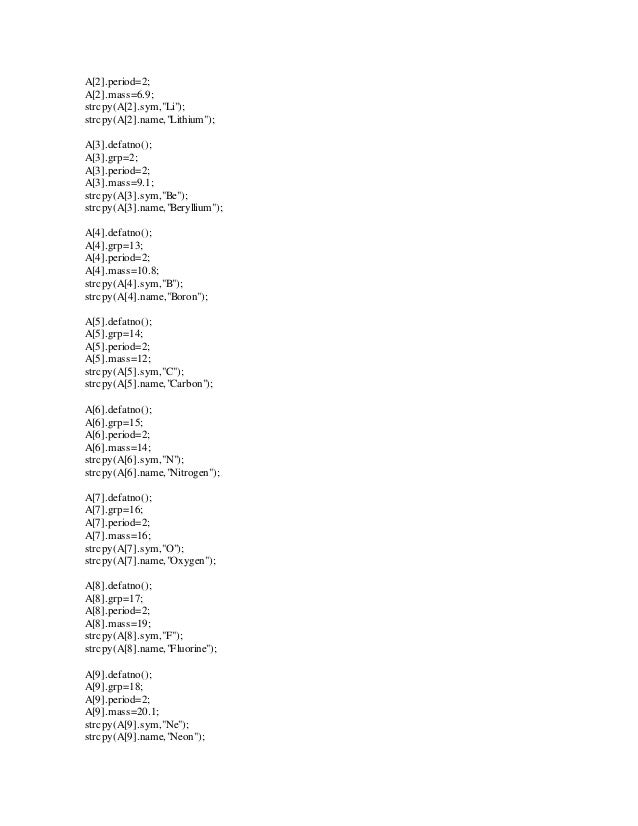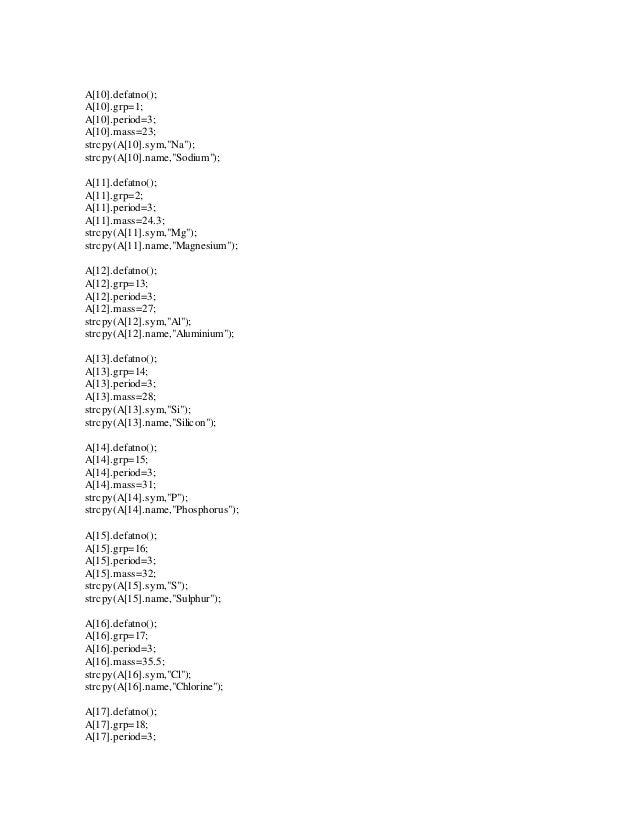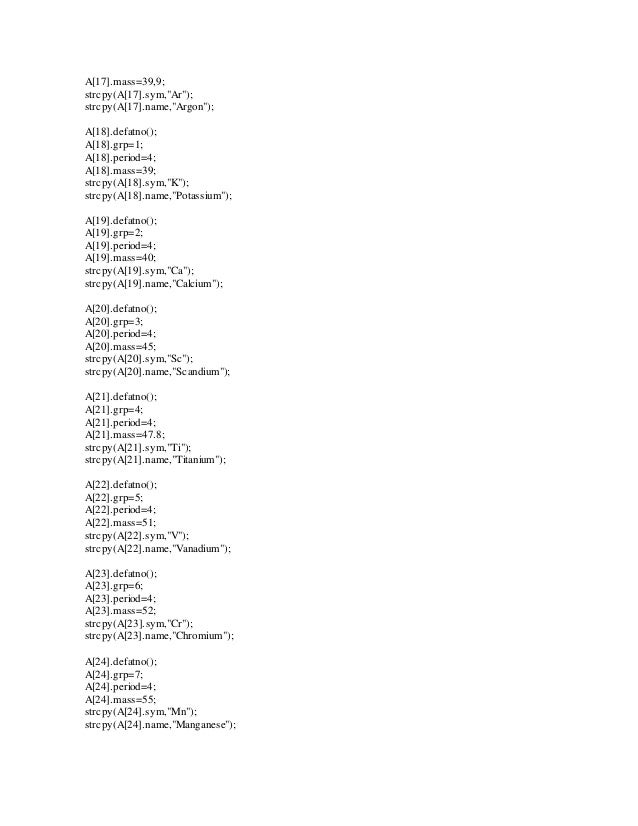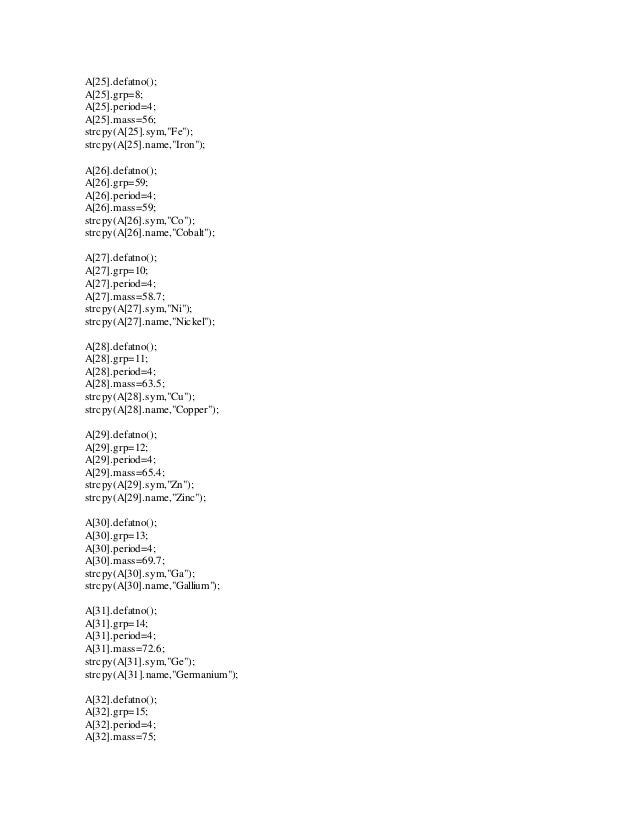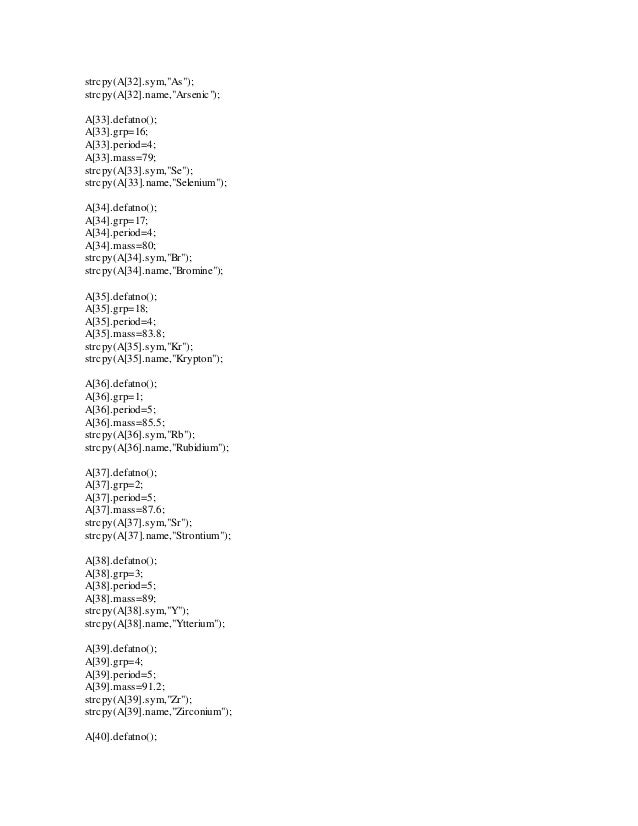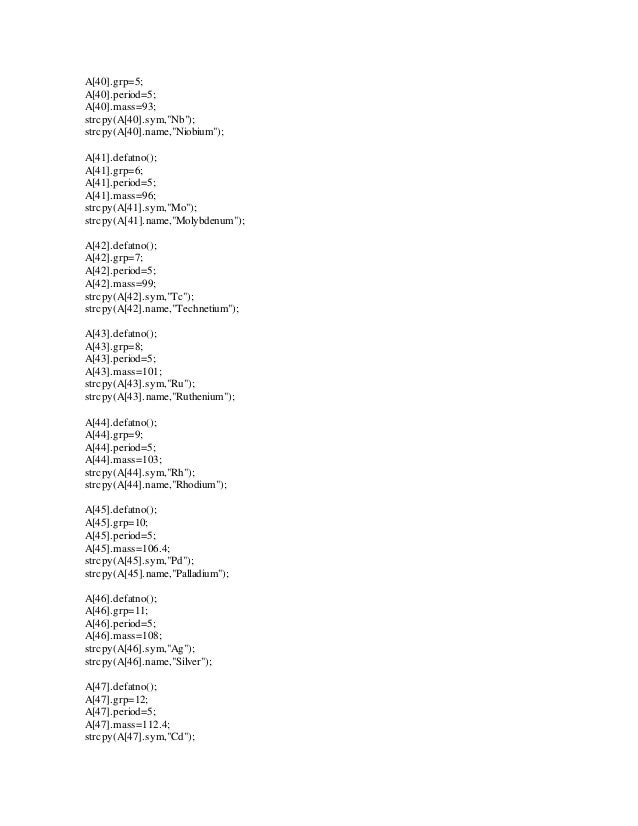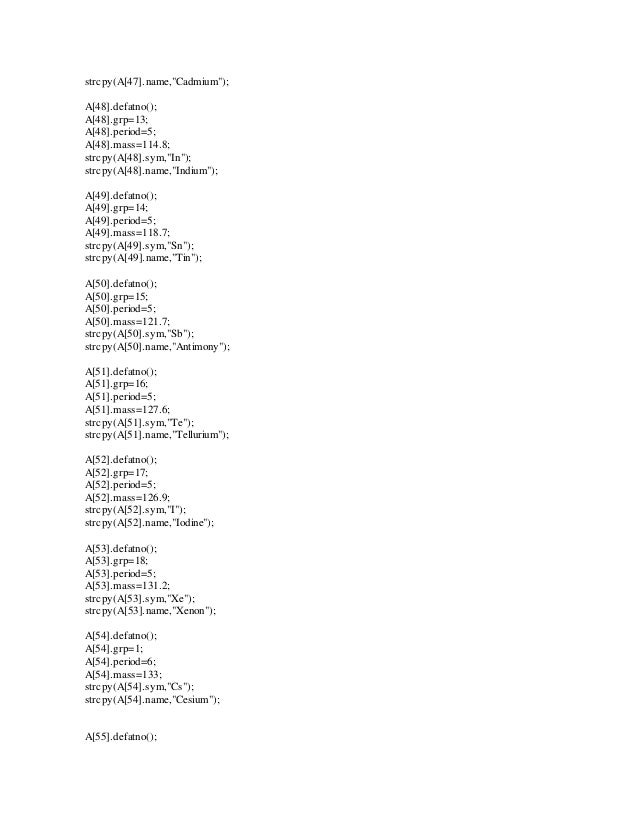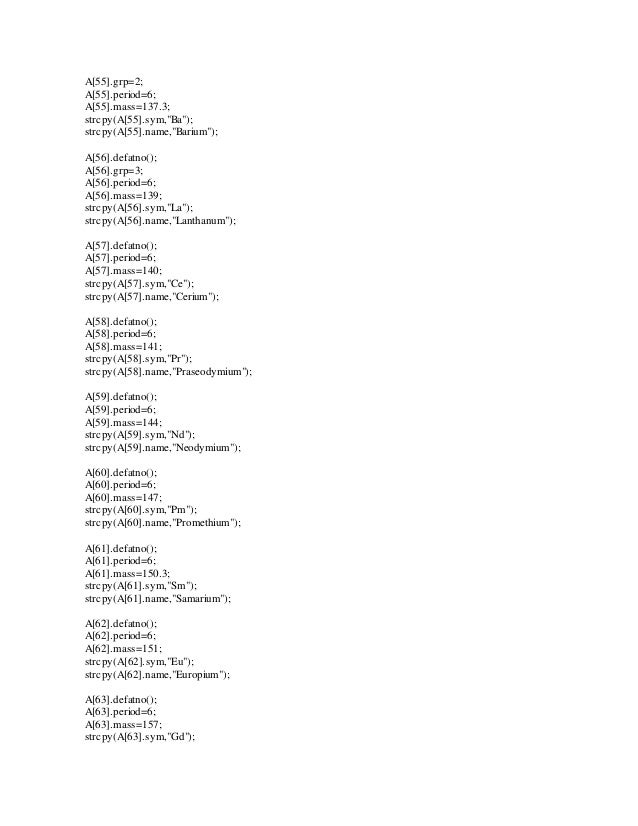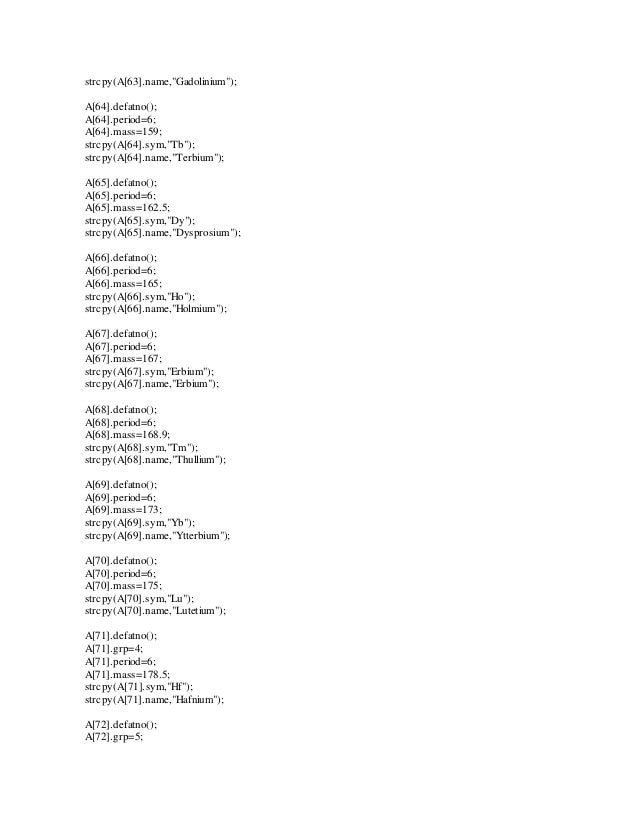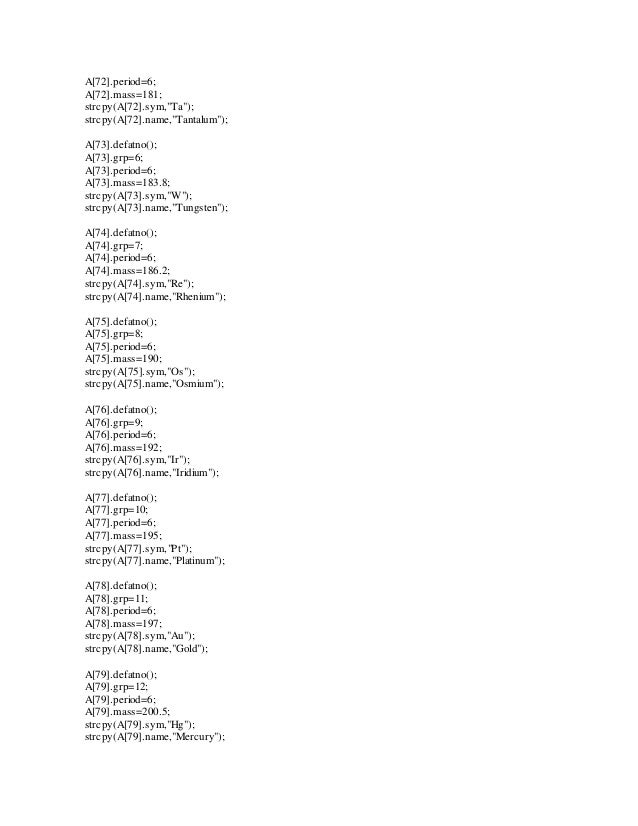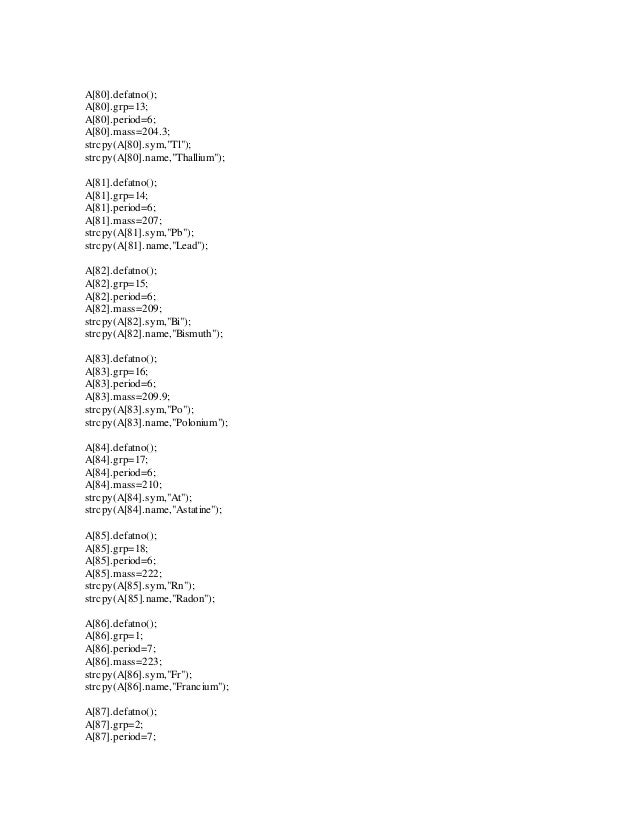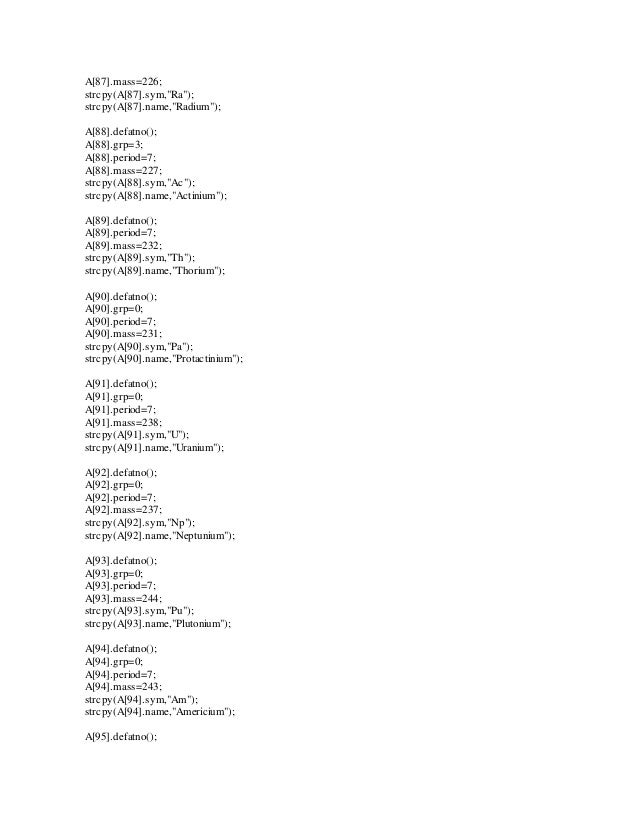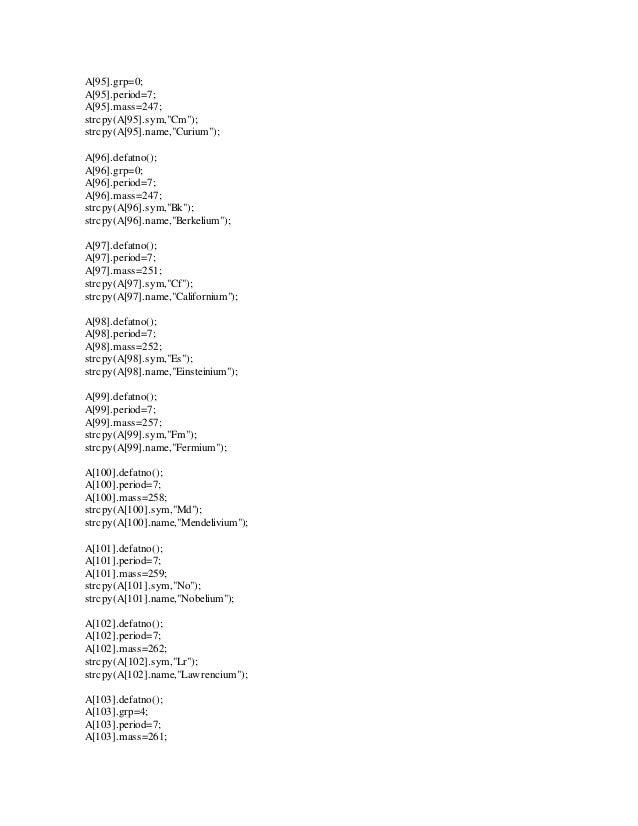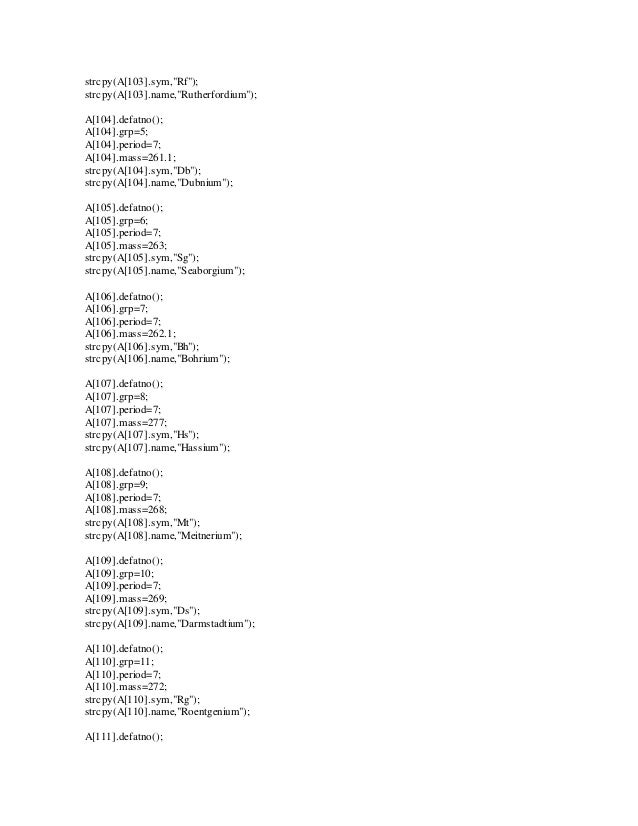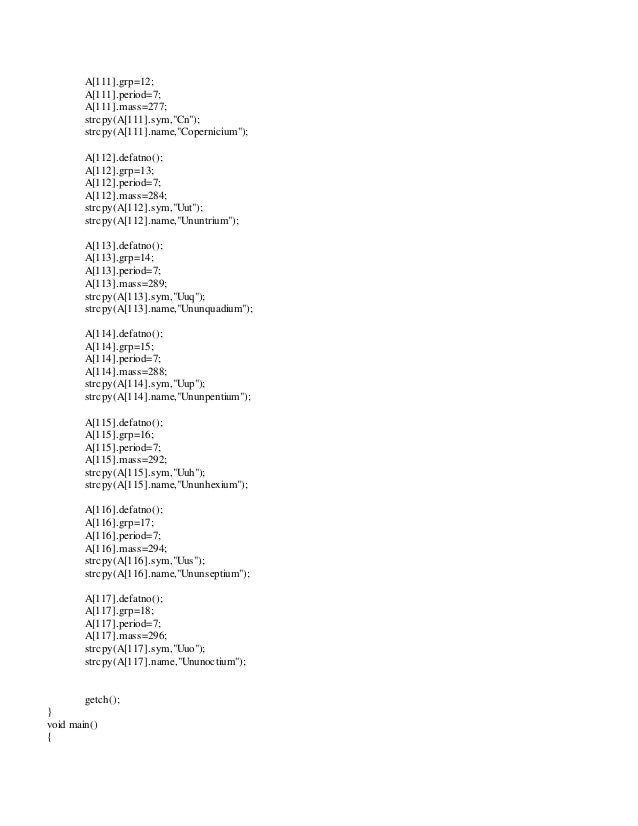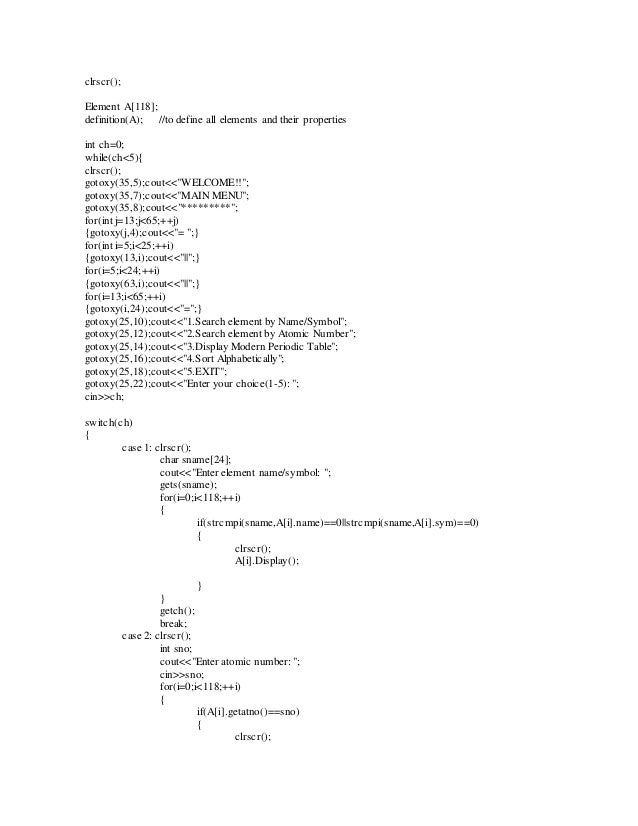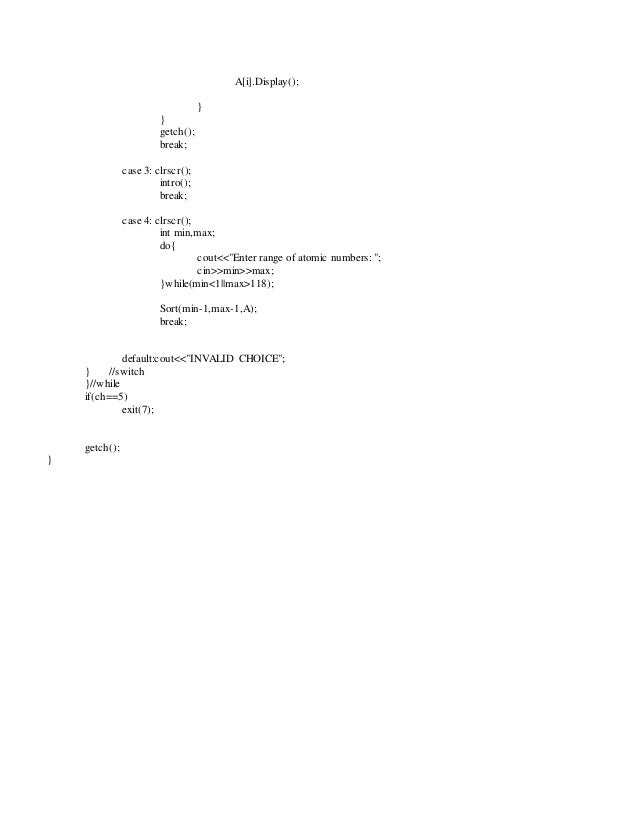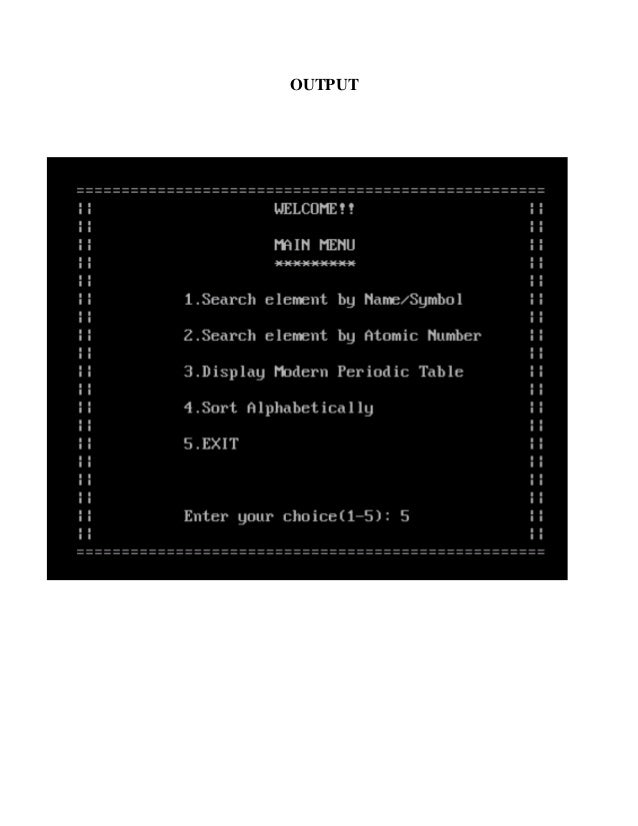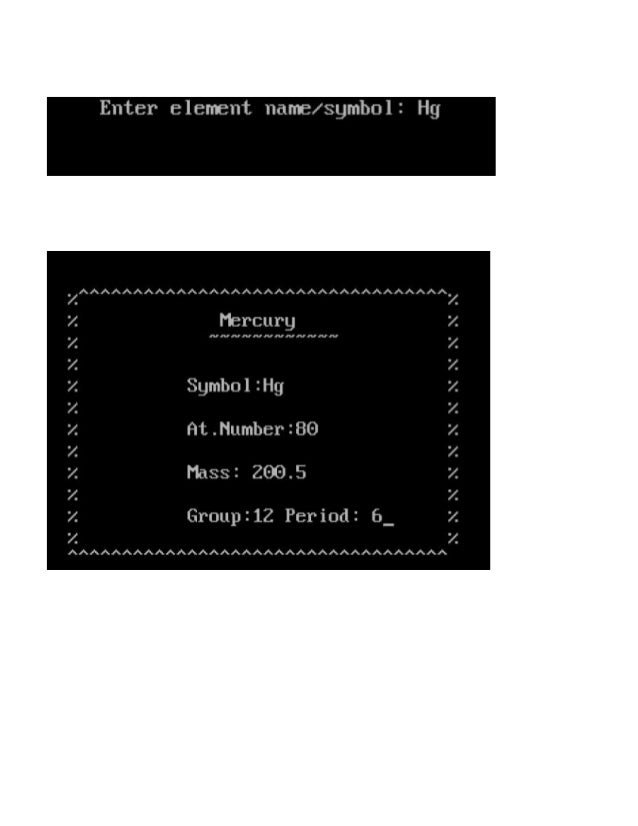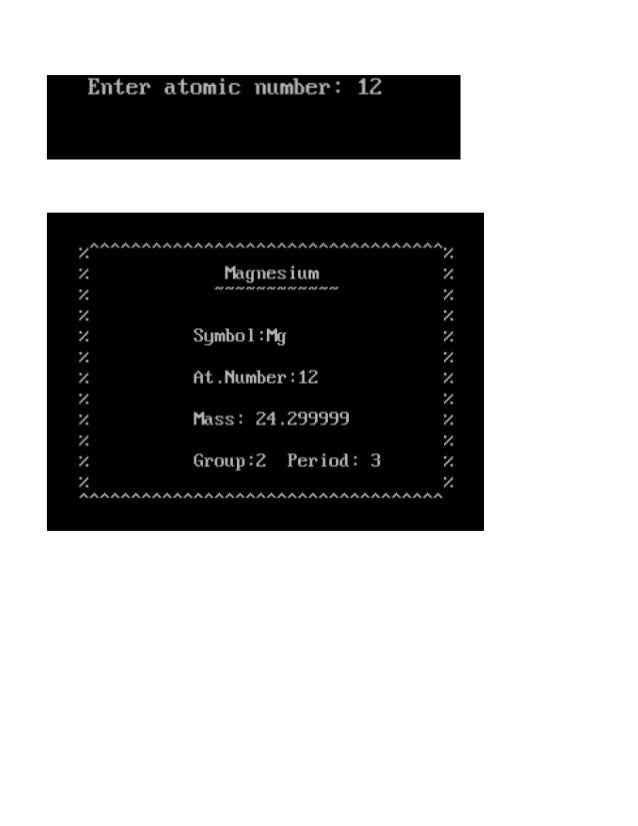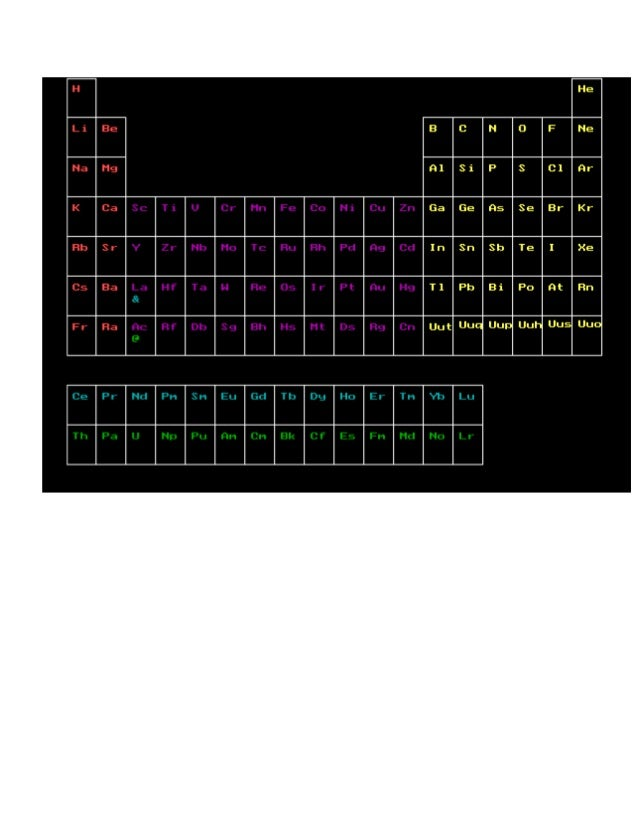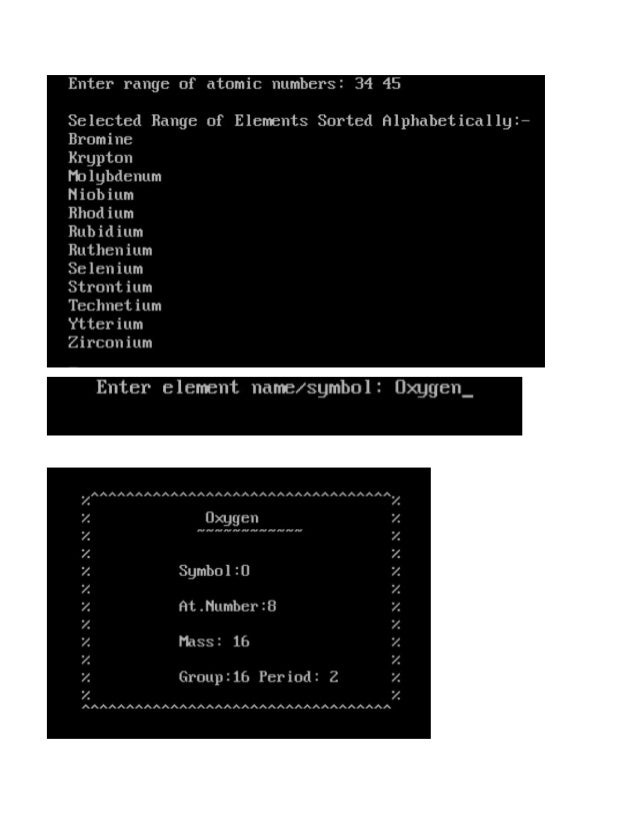Upcoming SlideShare
×
• Full Name
Comment goes here.

Are you sure you want to Yes No• Be the first to comment

### CBSE Computer Project for Class 12 ( C++)

1. 1. CONTENTS  HEADER FILES AND FUNCTIONS  WORKING DESCRIPTION  SOURCE CODE  OUTPUT
2. 2. WORKING DESCRIPTION This program is based on the Modern Periodic Table of Elements. It showcases all the elements and their basic properties including name, symbol, atomic number, atomic mass, group and period. This program contains 5 options: 1) Search element by name or symbol 2) Search element by atomic number 3) Display Modern Periodic Table 4) Sort elements alphabetically (Using Bubble Sort Technique) 5) Exit
3. 3. HEADER FILES AND FUNCTIONS HEADER FILES: 1) iostream.h – For cin, cout 2) conio.h – For console input output functions 3) stdio.h – For standard input output functions 4) stdlib.h – For exit function 5) graphics.h – For graphic related functions 6) string.h – For string manipulation function INBUILT FUNCTIONS: 1) gotoxy() 2) strcmpi() 3) strcpy() 4) clrscr() 5) getch() 6) gets() 7) exit() 8) initgraph() 9) line() 10) outtextxy() 11) rectangle() 12) setcolor() 13) getmaxx() 14) getmaxy() 15) closegraph()
4. 4. SOURCE CODE /* Developed By: KARAN SINGH BORA Class : 12-B */ #include<iostream.h> #include<string.h> #include<stdio.h> #include<stdlib.h> #include<graphics.h> #include<conio.h> class Element { int atno; public: int grp,period; float mass; char name; char sym; Element() { atno=period=1; grp=0; mass=0; strcpy(name,"Hydrogen"); strcpy(sym,"H"); } void defatno(); int getatno(){ return atno;}; void Display(); }; void Element::defatno() { static int x=1; atno=x; ++x; } void Element::Display() { for(int k=20;k<55;++k) { gotoxy(k,8);cout<<"^";} for(k=8;k<20;++k) { gotoxy(20,k);cout<<"%";} for(k=20;k<55;++k) { gotoxy(k,20);cout<<"^";} for(k=8;k<20;++k) { gotoxy(55,k);cout<<"%";}
5. 5. gotoxy(34,9);puts(name); gotoxy(33,10);cout<<"~~~~~~~~~~~~"; gotoxy(31,12);cout<<"Symbol:"<<sym; gotoxy(31,14);cout<<"At.Number:"<<atno; gotoxy(31,16);cout<<"Mass: "<<mass; gotoxy(31,18);cout<<"Group:"<<grp; gotoxy(40,18);cout<<"Period: "<<period; getch(); } void Sort(int a,int b,Element A) { for(int i=a-a;i<=b-a;++i) { for(int j=a-a;j<b-a-i;++j) { if(strcmpi(A[a+j].name,A[a+j+1].name)>0) { char temp; strcpy(temp,A[j+a].name); strcpy(A[j+a].name,A[j+1+a].name); strcpy(A[j+1+a].name,temp); } } } cout<<"nSelected Range of Elements Sorted Alphabetically:-n"; for(int k=a;k<=b;++k) { cout<<A[k].name<<endl; } getch(); } void intro() { clrscr(); int gr=0,gm=DETECT; initgraph(&gr,&gm,"C:TCBGI"); int x=getmaxx(); int y=getmaxy(); //main table line(1,1,1,280); line(30,1,30,280); line(60,40,60,280); line(90,120,90,280); line(120,120,120,280); line(150,120,150,280); line(180,120,180,280); line(210,120,210,280); line(240,120,240,280); line(270,120,270,280); line(300,120,300,280); line(330,120,330,280);
6. 6. line(360,40,360,280); line(390,40,390,280); line(420,40,420,280); line(450,40,450,280); line(480,40,480,280); line(510,1,510,280); line(540,1,540,280); line(1,1,30,1);line(510,1,540,1);//top line(1,280,540,280);//bottom line(1,40,60,40);line(360,40,540,40);// 1 period line(1,80,60,80);line(360,80,540,80);//2 period line(1,120,540,120);line(360,120,540,120);//3 period line(1,160,540,160); line(1,200,540,200); line(1,240,540,240); rectangle(1,310,420,390);//lanthanoids line(1,350,420,350);//partition // f-block partitions line(30,310,30,390); line(60,310,60,390); line(90,310,90,390); line(120,310,120,390); line(150,310,150,390); line(180,310,180,390); line(210,310,210,390); line(240,310,240,390); line(270,310,270,390); line(300,310,300,390); line(330,310,330,390); line(360,310,360,390); line(390,310,390,390); setcolor(CYAN); //lanthanoids outtextxy(7,318,"Ce"); outtextxy(37,318,"Pr"); outtextxy(67,318,"Nd"); outtextxy(97,318,"Pm"); outtextxy(127,318,"Sm"); outtextxy(157,318,"Eu"); outtextxy(187,318,"Gd"); outtextxy(217,318,"Tb"); outtextxy(247,318,"Dy"); outtextxy(277,318,"Ho"); outtextxy(307,318,"Er"); outtextxy(337,318,"Tm"); outtextxy(367,318,"Yb"); outtextxy(397,318,"Lu"); //actinoids
7. 7. setcolor(GREEN); outtextxy(7,358,"Th"); outtextxy(37,358,"Pa"); outtextxy(67,358,"U"); outtextxy(97,358,"Np"); outtextxy(127,358,"Pu"); outtextxy(157,358,"Am"); outtextxy(187,358,"Cm"); outtextxy(217,358,"Bk"); outtextxy(247,358,"Cf"); outtextxy(277,358,"Es"); outtextxy(307,358,"Fm"); outtextxy(337,358,"Md"); outtextxy(367,358,"No"); outtextxy(397,358,"Lr"); setcolor(12); outtextxy(7,8,"H"); outtextxy(7,48,"Li"); outtextxy(7,88,"Na"); outtextxy(7,128,"K"); outtextxy(7,168,"Rb"); outtextxy(7,208,"Cs"); outtextxy(7,248,"Fr"); outtextxy(37,48,"Be"); outtextxy(37,88,"Mg"); outtextxy(37,128,"Ca"); outtextxy(37,168,"Sr"); outtextxy(37,208,"Ba"); outtextxy(37,248,"Ra"); setcolor(MAGENTA); outtextxy(67,128,"Sc"); outtextxy(67,168,"Y"); outtextxy(67,208,"La"); outtextxy(67,248,"Ac"); setcolor(CYAN); outtextxy(67,220,"&"); setcolor(GREEN); outtextxy(67,260,"@"); setcolor(MAGENTA); outtextxy(97,128,"Ti"); outtextxy(97,168,"Zr"); outtextxy(97,208,"Hf"); outtextxy(97,248,"Rf"); outtextxy(127,128,"V"); outtextxy(127,168,"Nb"); outtextxy(127,208,"Ta"); outtextxy(127,248,"Db");
8. 8. outtextxy(157,128,"Cr"); outtextxy(157,168,"Mo"); outtextxy(157,208,"W"); outtextxy(157,248,"Sg"); outtextxy(187,128,"Mn"); outtextxy(187,168,"Tc"); outtextxy(187,208,"Re"); outtextxy(187,248,"Bh"); outtextxy(217,128,"Fe"); outtextxy(217,168,"Ru"); outtextxy(217,208,"Os"); outtextxy(217,248,"Hs"); outtextxy(247,128,"Co"); outtextxy(247,168,"Rh"); outtextxy(247,208,"Ir"); outtextxy(247,248,"Mt"); outtextxy(277,128,"Ni"); outtextxy(277,168,"Pd"); outtextxy(277,208,"Pt"); outtextxy(277,248,"Ds"); outtextxy(307,128,"Cu"); outtextxy(307,168,"Ag"); outtextxy(307,208,"Au"); outtextxy(307,248,"Rg"); outtextxy(337,128,"Zn"); outtextxy(337,168,"Cd"); outtextxy(337,208,"Hg"); outtextxy(337,248,"Cn"); setcolor(YELLOW); outtextxy(367,48,"B"); outtextxy(367,88,"Al"); outtextxy(367,128,"Ga"); outtextxy(367,168,"In"); outtextxy(367,208,"Tl"); outtextxy(367,248,"Uut"); outtextxy(397,48,"C"); outtextxy(397,88,"Si"); outtextxy(397,128,"Ge"); outtextxy(397,168,"Sn"); outtextxy(397,208,"Pb"); outtextxy(397,246,"Uuq"); outtextxy(427,48,"N"); outtextxy(427,88,"P"); outtextxy(427,128,"As");
9. 9. outtextxy(427,168,"Sb"); outtextxy(427,208,"Bi"); outtextxy(427,246,"Uup"); outtextxy(457,48,"O"); outtextxy(457,88,"S"); outtextxy(457,128,"Se"); outtextxy(457,168,"Te"); outtextxy(457,208,"Po"); outtextxy(457,246,"Uuh"); outtextxy(487,48,"F"); outtextxy(487,88,"Cl"); outtextxy(487,128,"Br"); outtextxy(487,168,"I"); outtextxy(487,208,"At"); outtextxy(487,245,"Uus"); outtextxy(517,8,"He"); outtextxy(517,48,"Ne"); outtextxy(517,88,"Ar"); outtextxy(517,128,"Kr"); outtextxy(517,168,"Xe"); outtextxy(517,208,"Rn"); outtextxy(517,245,"Uuo"); getch(); closegraph(); //end of intro page graphics } void definition(Element A) { clrscr(); A.defatno(); A.grp=1; A.period=1; A.mass=1; strcpy(A.sym,"H"); strcpy(A.name,"Hydrogen"); A.defatno(); A.grp=18; A.period=1; A.mass=4; strcpy(A.sym,"He"); strcpy(A.name,"Helium"); A.defatno(); A.grp=1;
10. 10. A.period=2; A.mass=6.9; strcpy(A.sym,"Li"); strcpy(A.name,"Lithium"); A.defatno(); A.grp=2; A.period=2; A.mass=9.1; strcpy(A.sym,"Be"); strcpy(A.name,"Beryllium"); A.defatno(); A.grp=13; A.period=2; A.mass=10.8; strcpy(A.sym,"B"); strcpy(A.name,"Boron"); A.defatno(); A.grp=14; A.period=2; A.mass=12; strcpy(A.sym,"C"); strcpy(A.name,"Carbon"); A.defatno(); A.grp=15; A.period=2; A.mass=14; strcpy(A.sym,"N"); strcpy(A.name,"Nitrogen"); A.defatno(); A.grp=16; A.period=2; A.mass=16; strcpy(A.sym,"O"); strcpy(A.name,"Oxygen"); A.defatno(); A.grp=17; A.period=2; A.mass=19; strcpy(A.sym,"F"); strcpy(A.name,"Fluorine"); A.defatno(); A.grp=18; A.period=2; A.mass=20.1; strcpy(A.sym,"Ne"); strcpy(A.name,"Neon");
11. 11. A.defatno(); A.grp=1; A.period=3; A.mass=23; strcpy(A.sym,"Na"); strcpy(A.name,"Sodium"); A.defatno(); A.grp=2; A.period=3; A.mass=24.3; strcpy(A.sym,"Mg"); strcpy(A.name,"Magnesium"); A.defatno(); A.grp=13; A.period=3; A.mass=27; strcpy(A.sym,"Al"); strcpy(A.name,"Aluminium"); A.defatno(); A.grp=14; A.period=3; A.mass=28; strcpy(A.sym,"Si"); strcpy(A.name,"Silicon"); A.defatno(); A.grp=15; A.period=3; A.mass=31; strcpy(A.sym,"P"); strcpy(A.name,"Phosphorus"); A.defatno(); A.grp=16; A.period=3; A.mass=32; strcpy(A.sym,"S"); strcpy(A.name,"Sulphur"); A.defatno(); A.grp=17; A.period=3; A.mass=35.5; strcpy(A.sym,"Cl"); strcpy(A.name,"Chlorine"); A.defatno(); A.grp=18; A.period=3;
12. 12. A.mass=39,9; strcpy(A.sym,"Ar"); strcpy(A.name,"Argon"); A.defatno(); A.grp=1; A.period=4; A.mass=39; strcpy(A.sym,"K"); strcpy(A.name,"Potassium"); A.defatno(); A.grp=2; A.period=4; A.mass=40; strcpy(A.sym,"Ca"); strcpy(A.name,"Calcium"); A.defatno(); A.grp=3; A.period=4; A.mass=45; strcpy(A.sym,"Sc"); strcpy(A.name,"Scandium"); A.defatno(); A.grp=4; A.period=4; A.mass=47.8; strcpy(A.sym,"Ti"); strcpy(A.name,"Titanium"); A.defatno(); A.grp=5; A.period=4; A.mass=51; strcpy(A.sym,"V"); strcpy(A.name,"Vanadium"); A.defatno(); A.grp=6; A.period=4; A.mass=52; strcpy(A.sym,"Cr"); strcpy(A.name,"Chromium"); A.defatno(); A.grp=7; A.period=4; A.mass=55; strcpy(A.sym,"Mn"); strcpy(A.name,"Manganese");
13. 13. A.defatno(); A.grp=8; A.period=4; A.mass=56; strcpy(A.sym,"Fe"); strcpy(A.name,"Iron"); A.defatno(); A.grp=59; A.period=4; A.mass=59; strcpy(A.sym,"Co"); strcpy(A.name,"Cobalt"); A.defatno(); A.grp=10; A.period=4; A.mass=58.7; strcpy(A.sym,"Ni"); strcpy(A.name,"Nickel"); A.defatno(); A.grp=11; A.period=4; A.mass=63.5; strcpy(A.sym,"Cu"); strcpy(A.name,"Copper"); A.defatno(); A.grp=12; A.period=4; A.mass=65.4; strcpy(A.sym,"Zn"); strcpy(A.name,"Zinc"); A.defatno(); A.grp=13; A.period=4; A.mass=69.7; strcpy(A.sym,"Ga"); strcpy(A.name,"Gallium"); A.defatno(); A.grp=14; A.period=4; A.mass=72.6; strcpy(A.sym,"Ge"); strcpy(A.name,"Germanium"); A.defatno(); A.grp=15; A.period=4; A.mass=75;
14. 14. strcpy(A.sym,"As"); strcpy(A.name,"Arsenic"); A.defatno(); A.grp=16; A.period=4; A.mass=79; strcpy(A.sym,"Se"); strcpy(A.name,"Selenium"); A.defatno(); A.grp=17; A.period=4; A.mass=80; strcpy(A.sym,"Br"); strcpy(A.name,"Bromine"); A.defatno(); A.grp=18; A.period=4; A.mass=83.8; strcpy(A.sym,"Kr"); strcpy(A.name,"Krypton"); A.defatno(); A.grp=1; A.period=5; A.mass=85.5; strcpy(A.sym,"Rb"); strcpy(A.name,"Rubidium"); A.defatno(); A.grp=2; A.period=5; A.mass=87.6; strcpy(A.sym,"Sr"); strcpy(A.name,"Strontium"); A.defatno(); A.grp=3; A.period=5; A.mass=89; strcpy(A.sym,"Y"); strcpy(A.name,"Ytterium"); A.defatno(); A.grp=4; A.period=5; A.mass=91.2; strcpy(A.sym,"Zr"); strcpy(A.name,"Zirconium"); A.defatno();
15. 15. A.grp=5; A.period=5; A.mass=93; strcpy(A.sym,"Nb"); strcpy(A.name,"Niobium"); A.defatno(); A.grp=6; A.period=5; A.mass=96; strcpy(A.sym,"Mo"); strcpy(A.name,"Molybdenum"); A.defatno(); A.grp=7; A.period=5; A.mass=99; strcpy(A.sym,"Tc"); strcpy(A.name,"Technetium"); A.defatno(); A.grp=8; A.period=5; A.mass=101; strcpy(A.sym,"Ru"); strcpy(A.name,"Ruthenium"); A.defatno(); A.grp=9; A.period=5; A.mass=103; strcpy(A.sym,"Rh"); strcpy(A.name,"Rhodium"); A.defatno(); A.grp=10; A.period=5; A.mass=106.4; strcpy(A.sym,"Pd"); strcpy(A.name,"Palladium"); A.defatno(); A.grp=11; A.period=5; A.mass=108; strcpy(A.sym,"Ag"); strcpy(A.name,"Silver"); A.defatno(); A.grp=12; A.period=5; A.mass=112.4; strcpy(A.sym,"Cd");
16. 16. strcpy(A.name,"Cadmium"); A.defatno(); A.grp=13; A.period=5; A.mass=114.8; strcpy(A.sym,"In"); strcpy(A.name,"Indium"); A.defatno(); A.grp=14; A.period=5; A.mass=118.7; strcpy(A.sym,"Sn"); strcpy(A.name,"Tin"); A.defatno(); A.grp=15; A.period=5; A.mass=121.7; strcpy(A.sym,"Sb"); strcpy(A.name,"Antimony"); A.defatno(); A.grp=16; A.period=5; A.mass=127.6; strcpy(A.sym,"Te"); strcpy(A.name,"Tellurium"); A.defatno(); A.grp=17; A.period=5; A.mass=126.9; strcpy(A.sym,"I"); strcpy(A.name,"Iodine"); A.defatno(); A.grp=18; A.period=5; A.mass=131.2; strcpy(A.sym,"Xe"); strcpy(A.name,"Xenon"); A.defatno(); A.grp=1; A.period=6; A.mass=133; strcpy(A.sym,"Cs"); strcpy(A.name,"Cesium"); A.defatno();
17. 17. A.grp=2; A.period=6; A.mass=137.3; strcpy(A.sym,"Ba"); strcpy(A.name,"Barium"); A.defatno(); A.grp=3; A.period=6; A.mass=139; strcpy(A.sym,"La"); strcpy(A.name,"Lanthanum"); A.defatno(); A.period=6; A.mass=140; strcpy(A.sym,"Ce"); strcpy(A.name,"Cerium"); A.defatno(); A.period=6; A.mass=141; strcpy(A.sym,"Pr"); strcpy(A.name,"Praseodymium"); A.defatno(); A.period=6; A.mass=144; strcpy(A.sym,"Nd"); strcpy(A.name,"Neodymium"); A.defatno(); A.period=6; A.mass=147; strcpy(A.sym,"Pm"); strcpy(A.name,"Promethium"); A.defatno(); A.period=6; A.mass=150.3; strcpy(A.sym,"Sm"); strcpy(A.name,"Samarium"); A.defatno(); A.period=6; A.mass=151; strcpy(A.sym,"Eu"); strcpy(A.name,"Europium"); A.defatno(); A.period=6; A.mass=157; strcpy(A.sym,"Gd");
18. 18. strcpy(A.name,"Gadolinium"); A.defatno(); A.period=6; A.mass=159; strcpy(A.sym,"Tb"); strcpy(A.name,"Terbium"); A.defatno(); A.period=6; A.mass=162.5; strcpy(A.sym,"Dy"); strcpy(A.name,"Dysprosium"); A.defatno(); A.period=6; A.mass=165; strcpy(A.sym,"Ho"); strcpy(A.name,"Holmium"); A.defatno(); A.period=6; A.mass=167; strcpy(A.sym,"Erbium"); strcpy(A.name,"Erbium"); A.defatno(); A.period=6; A.mass=168.9; strcpy(A.sym,"Tm"); strcpy(A.name,"Thullium"); A.defatno(); A.period=6; A.mass=173; strcpy(A.sym,"Yb"); strcpy(A.name,"Ytterbium"); A.defatno(); A.period=6; A.mass=175; strcpy(A.sym,"Lu"); strcpy(A.name,"Lutetium"); A.defatno(); A.grp=4; A.period=6; A.mass=178.5; strcpy(A.sym,"Hf"); strcpy(A.name,"Hafnium"); A.defatno(); A.grp=5;
19. 19. A.period=6; A.mass=181; strcpy(A.sym,"Ta"); strcpy(A.name,"Tantalum"); A.defatno(); A.grp=6; A.period=6; A.mass=183.8; strcpy(A.sym,"W"); strcpy(A.name,"Tungsten"); A.defatno(); A.grp=7; A.period=6; A.mass=186.2; strcpy(A.sym,"Re"); strcpy(A.name,"Rhenium"); A.defatno(); A.grp=8; A.period=6; A.mass=190; strcpy(A.sym,"Os"); strcpy(A.name,"Osmium"); A.defatno(); A.grp=9; A.period=6; A.mass=192; strcpy(A.sym,"Ir"); strcpy(A.name,"Iridium"); A.defatno(); A.grp=10; A.period=6; A.mass=195; strcpy(A.sym,"Pt"); strcpy(A.name,"Platinum"); A.defatno(); A.grp=11; A.period=6; A.mass=197; strcpy(A.sym,"Au"); strcpy(A.name,"Gold"); A.defatno(); A.grp=12; A.period=6; A.mass=200.5; strcpy(A.sym,"Hg"); strcpy(A.name,"Mercury");
20. 20. A.defatno(); A.grp=13; A.period=6; A.mass=204.3; strcpy(A.sym,"Tl"); strcpy(A.name,"Thallium"); A.defatno(); A.grp=14; A.period=6; A.mass=207; strcpy(A.sym,"Pb"); strcpy(A.name,"Lead"); A.defatno(); A.grp=15; A.period=6; A.mass=209; strcpy(A.sym,"Bi"); strcpy(A.name,"Bismuth"); A.defatno(); A.grp=16; A.period=6; A.mass=209.9; strcpy(A.sym,"Po"); strcpy(A.name,"Polonium"); A.defatno(); A.grp=17; A.period=6; A.mass=210; strcpy(A.sym,"At"); strcpy(A.name,"Astatine"); A.defatno(); A.grp=18; A.period=6; A.mass=222; strcpy(A.sym,"Rn"); strcpy(A.name,"Radon"); A.defatno(); A.grp=1; A.period=7; A.mass=223; strcpy(A.sym,"Fr"); strcpy(A.name,"Francium"); A.defatno(); A.grp=2; A.period=7;
21. 21. A.mass=226; strcpy(A.sym,"Ra"); strcpy(A.name,"Radium"); A.defatno(); A.grp=3; A.period=7; A.mass=227; strcpy(A.sym,"Ac"); strcpy(A.name,"Actinium"); A.defatno(); A.period=7; A.mass=232; strcpy(A.sym,"Th"); strcpy(A.name,"Thorium"); A.defatno(); A.grp=0; A.period=7; A.mass=231; strcpy(A.sym,"Pa"); strcpy(A.name,"Protactinium"); A.defatno(); A.grp=0; A.period=7; A.mass=238; strcpy(A.sym,"U"); strcpy(A.name,"Uranium"); A.defatno(); A.grp=0; A.period=7; A.mass=237; strcpy(A.sym,"Np"); strcpy(A.name,"Neptunium"); A.defatno(); A.grp=0; A.period=7; A.mass=244; strcpy(A.sym,"Pu"); strcpy(A.name,"Plutonium"); A.defatno(); A.grp=0; A.period=7; A.mass=243; strcpy(A.sym,"Am"); strcpy(A.name,"Americium"); A.defatno();
22. 22. A.grp=0; A.period=7; A.mass=247; strcpy(A.sym,"Cm"); strcpy(A.name,"Curium"); A.defatno(); A.grp=0; A.period=7; A.mass=247; strcpy(A.sym,"Bk"); strcpy(A.name,"Berkelium"); A.defatno(); A.period=7; A.mass=251; strcpy(A.sym,"Cf"); strcpy(A.name,"Californium"); A.defatno(); A.period=7; A.mass=252; strcpy(A.sym,"Es"); strcpy(A.name,"Einsteinium"); A.defatno(); A.period=7; A.mass=257; strcpy(A.sym,"Fm"); strcpy(A.name,"Fermium"); A.defatno(); A.period=7; A.mass=258; strcpy(A.sym,"Md"); strcpy(A.name,"Mendelivium"); A.defatno(); A.period=7; A.mass=259; strcpy(A.sym,"No"); strcpy(A.name,"Nobelium"); A.defatno(); A.period=7; A.mass=262; strcpy(A.sym,"Lr"); strcpy(A.name,"Lawrencium"); A.defatno(); A.grp=4; A.period=7; A.mass=261;
23. 23. strcpy(A.sym,"Rf"); strcpy(A.name,"Rutherfordium"); A.defatno(); A.grp=5; A.period=7; A.mass=261.1; strcpy(A.sym,"Db"); strcpy(A.name,"Dubnium"); A.defatno(); A.grp=6; A.period=7; A.mass=263; strcpy(A.sym,"Sg"); strcpy(A.name,"Seaborgium"); A.defatno(); A.grp=7; A.period=7; A.mass=262.1; strcpy(A.sym,"Bh"); strcpy(A.name,"Bohrium"); A.defatno(); A.grp=8; A.period=7; A.mass=277; strcpy(A.sym,"Hs"); strcpy(A.name,"Hassium"); A.defatno(); A.grp=9; A.period=7; A.mass=268; strcpy(A.sym,"Mt"); strcpy(A.name,"Meitnerium"); A.defatno(); A.grp=10; A.period=7; A.mass=269; strcpy(A.sym,"Ds"); strcpy(A.name,"Darmstadtium"); A.defatno(); A.grp=11; A.period=7; A.mass=272; strcpy(A.sym,"Rg"); strcpy(A.name,"Roentgenium"); A.defatno();
24. 24. A.grp=12; A.period=7; A.mass=277; strcpy(A.sym,"Cn"); strcpy(A.name,"Copernicium"); A.defatno(); A.grp=13; A.period=7; A.mass=284; strcpy(A.sym,"Uut"); strcpy(A.name,"Ununtrium"); A.defatno(); A.grp=14; A.period=7; A.mass=289; strcpy(A.sym,"Uuq"); strcpy(A.name,"Ununquadium"); A.defatno(); A.grp=15; A.period=7; A.mass=288; strcpy(A.sym,"Uup"); strcpy(A.name,"Ununpentium"); A.defatno(); A.grp=16; A.period=7; A.mass=292; strcpy(A.sym,"Uuh"); strcpy(A.name,"Ununhexium"); A.defatno(); A.grp=17; A.period=7; A.mass=294; strcpy(A.sym,"Uus"); strcpy(A.name,"Ununseptium"); A.defatno(); A.grp=18; A.period=7; A.mass=296; strcpy(A.sym,"Uuo"); strcpy(A.name,"Ununoctium"); getch(); } void main() {
25. 25. clrscr(); Element A; definition(A); //to define all elements and their properties int ch=0; while(ch<5){ clrscr(); gotoxy(35,5);cout<<"WELCOME!!"; gotoxy(35,7);cout<<"MAIN MENU"; gotoxy(35,8);cout<<"*********"; for(int j=13;j<65;++j) {gotoxy(j,4);cout<<"= ";} for(int i=5;i<25;++i) {gotoxy(13,i);cout<<"||";} for(i=5;i<24;++i) {gotoxy(63,i);cout<<"||";} for(i=13;i<65;++i) {gotoxy(i,24);cout<<"=";} gotoxy(25,10);cout<<"1.Search element by Name/Symbol"; gotoxy(25,12);cout<<"2.Search element by Atomic Number"; gotoxy(25,14);cout<<"3.Display Modern Periodic Table"; gotoxy(25,16);cout<<"4.Sort Alphabetically"; gotoxy(25,18);cout<<"5.EXIT"; gotoxy(25,22);cout<<"Enter your choice(1-5):"; cin>>ch; switch(ch) { case 1: clrscr(); char sname; cout<<"Enter element name/symbol: "; gets(sname); for(i=0;i<118;++i) { if(strcmpi(sname,A[i].name)==0||strcmpi(sname,A[i].sym)==0) { clrscr(); A[i].Display(); } } getch(); break; case 2: clrscr(); int sno; cout<<"Enter atomic number: "; cin>>sno; for(i=0;i<118;++i) { if(A[i].getatno()==sno) { clrscr();
26. 26. A[i].Display(); } } getch(); break; case 3: clrscr(); intro(); break; case 4: clrscr(); int min,max; do{ cout<<"Enter range of atomic numbers:"; cin>>min>>max; }while(min<1||max>118); Sort(min-1,max-1,A); break; default:cout<<"INVALID CHOICE"; } //switch }//while if(ch==5) exit(7); getch(); }
27. 27. OUTPUT

### Be the first to comment

•#### samdominic1

Oct. 19, 2017
•#### HarshitBisht2

Nov. 20, 2017
•#### mgrwl2

Dec. 12, 2017
•#### ShibaniDas2

Dec. 19, 2017
•Dec. 20, 2017
•#### Hemanttuteja

Jan. 10, 2018
•#### AmanKothari12

Jan. 14, 2018
•#### HarshShah492

Nov. 23, 2018
•#### shivanikumari47

Dec. 20, 2018
•#### JatinKshatriya1

Jan. 9, 2019
•#### DhiineshS

Jan. 17, 2019
•#### MrRex2

Jan. 24, 2019
•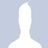#### nitinagarwal73113528

Oct. 11, 2019
•#### AnkitGupta1327

Nov. 23, 2019
•#### ManikandanathanKasini

Dec. 13, 2019
•#### SakshamSaxena30

Jan. 11, 2020
•#### anujkumar1152

Jan. 14, 2020
•#### pratyushagrawal10

Jan. 16, 2020
•#### ShubamRaina1

Mar. 5, 2020
•Dec. 9, 2020

Total views

13,587

On Slideshare

0

From embeds

0

Number of embeds

11

401

Shares

0